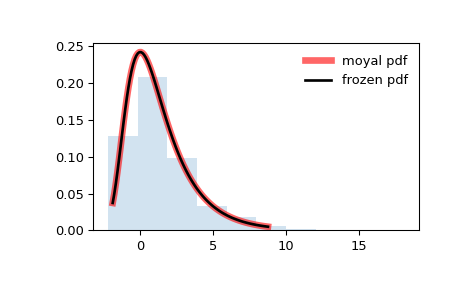# scipy.stats.moyal¶

scipy.stats.moyal(*args, **kwds) = <scipy.stats._continuous_distns.moyal_gen object>[source]

A Moyal continuous random variable.

As an instance of the rv_continuous class, moyal object inherits from it a collection of generic methods (see below for the full list), and completes them with details specific for this particular distribution.

Notes

The probability density function for moyal is:

$f(x) = \exp(-(x + \exp(-x))/2) / \sqrt{2\pi}$

for a real number $$x$$.

The probability density above is defined in the “standardized” form. To shift and/or scale the distribution use the loc and scale parameters. Specifically, moyal.pdf(x, loc, scale) is identically equivalent to moyal.pdf(y) / scale with y = (x - loc) / scale.

This distribution has utility in high-energy physics and radiation detection. It describes the energy loss of a charged relativistic particle due to ionization of the medium . It also provides an approximation for the Landau distribution. For an in depth description see . For additional description, see .

References

1

J.E. Moyal, “XXX. Theory of ionization fluctuations”, The London, Edinburgh, and Dublin Philosophical Magazine and Journal of Science, vol 46, 263-280, (1955). DOI:10.1080/14786440308521076 (gated)

2

G. Cordeiro et al., “The beta Moyal: a useful skew distribution”, International Journal of Research and Reviews in Applied Sciences, vol 10, 171-192, (2012). http://www.arpapress.com/Volumes/Vol10Issue2/IJRRAS_10_2_02.pdf

3

C. Walck, “Handbook on Statistical Distributions for Experimentalists; International Report SUF-PFY/96-01”, Chapter 26, University of Stockholm: Stockholm, Sweden, (2007). http://www.stat.rice.edu/~dobelman/textfiles/DistributionsHandbook.pdf

New in version 1.1.0.

Examples

>>> from scipy.stats import moyal
>>> import matplotlib.pyplot as plt
>>> fig, ax = plt.subplots(1, 1)


Calculate a few first moments:

>>> mean, var, skew, kurt = moyal.stats(moments='mvsk')


Display the probability density function (pdf):

>>> x = np.linspace(moyal.ppf(0.01),
...                 moyal.ppf(0.99), 100)
>>> ax.plot(x, moyal.pdf(x),
...        'r-', lw=5, alpha=0.6, label='moyal pdf')


Alternatively, the distribution object can be called (as a function) to fix the shape, location and scale parameters. This returns a “frozen” RV object holding the given parameters fixed.

Freeze the distribution and display the frozen pdf:

>>> rv = moyal()
>>> ax.plot(x, rv.pdf(x), 'k-', lw=2, label='frozen pdf')


Check accuracy of cdf and ppf:

>>> vals = moyal.ppf([0.001, 0.5, 0.999])
>>> np.allclose([0.001, 0.5, 0.999], moyal.cdf(vals))
True


Generate random numbers:

>>> r = moyal.rvs(size=1000)


And compare the histogram:

>>> ax.hist(r, density=True, histtype='stepfilled', alpha=0.2)
>>> ax.legend(loc='best', frameon=False)
>>> plt.show()Methods

 rvs(loc=0, scale=1, size=1, random_state=None) Random variates. pdf(x, loc=0, scale=1) Probability density function. logpdf(x, loc=0, scale=1) Log of the probability density function. cdf(x, loc=0, scale=1) Cumulative distribution function. logcdf(x, loc=0, scale=1) Log of the cumulative distribution function. sf(x, loc=0, scale=1) Survival function (also defined as 1 - cdf, but sf is sometimes more accurate). logsf(x, loc=0, scale=1) Log of the survival function. ppf(q, loc=0, scale=1) Percent point function (inverse of cdf — percentiles). isf(q, loc=0, scale=1) Inverse survival function (inverse of sf). moment(n, loc=0, scale=1) Non-central moment of order n stats(loc=0, scale=1, moments=’mv’) Mean(‘m’), variance(‘v’), skew(‘s’), and/or kurtosis(‘k’). entropy(loc=0, scale=1) (Differential) entropy of the RV. fit(data) Parameter estimates for generic data. See scipy.stats.rv_continuous.fit for detailed documentation of the keyword arguments. expect(func, args=(), loc=0, scale=1, lb=None, ub=None, conditional=False, **kwds) Expected value of a function (of one argument) with respect to the distribution. median(loc=0, scale=1) Median of the distribution. mean(loc=0, scale=1) Mean of the distribution. var(loc=0, scale=1) Variance of the distribution. std(loc=0, scale=1) Standard deviation of the distribution. interval(alpha, loc=0, scale=1) Endpoints of the range that contains alpha percent of the distribution

#### Previous topic

scipy.stats.mielke

#### Next topic

scipy.stats.nakagami